# Supporting the TEKS Mathematical Processes with Exemplars Performance Tasks and Rubric at the First Grade Level

Written By Exemplars Math Consultants: Deborah Armitage, M.Ed. and Dinah Chancellor, M.Ed.

###### Blog Series Overview:

Exemplars performance-based material is a supplemental resource that provides teachers with an effective way to implement the Math TEKS through problem solving. This blog represents Part 2 of a five-part series that features a problem-solving task linked to a Unit of Study for the Math TEKS and a student’s solution in grades K–5. Evidence of all seven Mathematical Process Standards will be exhibited by the end of the series.

The Exemplars Standards-Based Math Rubric allows teachers to examine student work against a set of analytic criteria that consists of the following categories: Problem Solving, Reasoning and Proof, Communication, Connections and Representation. There are four performance/achievement levels: Novice, Apprentice, Practitioner (meets the standard) and Expert. The Novice and Apprentice levels support a student’s progress towards being able to apply the criteria of a Practitioner and Expert. It is at these higher levels of achievement where support for the Mathematical Processes is found.

Exemplars problem-solving tasks provide students with an opportunity to apply their conceptual understanding of standards, mathematical processes and skills. Observing student anchor papers with assessment rationales that demonstrate the alignment between the Exemplars assessment rubric and the Math TEKS can be insightful for educators. Anchor papers and assessment rationales provide examples of what to look for in your own students’ work. Examples of Exemplars rubric criteria and the Mathematical Processes are embedded in the assessment rationales at the bottom of the page. The full version of our rubric may be accessed here. It is often helpful to have this in-hand while reviewing a piece of student work.

#### Blog 2: Observations at the First Grade Level

The second anchor paper and set of assessment rationales we’ll review in this series is taken from a first grade student’s solution for the task “A Birdbath.” In this piece, you’ll notice that the teacher has “scribed” the student’s oral explanation. This practice was also used with the Kindergarten task that was published in the first blog. Scribing allows teachers to fully capture the mathematical reasoning of early writers.

“A Birdbath” is one of a number of tasks aligned to the Strategies for Addition and Subtraction Unit designed by Exemplars for the new Math TEKS. This task could be used toward the end of the learning time allocated to this Unit. “A Birdbath” provides first grade students with an opportunity to apply different strategies to find the sum of addends six and 14 by decomposing six into five and one and decomposing 14 into 10 and four, or by finding the sum of six and four and adding that sum to 10. The student can use counters, ten frames, a Rekenrek, number lines or a tally chart to support her/his numerical thinking.

Leah counts the birds that came to her birdbath. In the morning, Leah counts six birds that came to her birdbath. In the afternoon, Leah counts fourteen birds that came to her birdbath. Leah says nineteen birds came to her birdbath. Is Leah correct? Show all of your mathematical thinking.

### Math TEKS Alignment:

###### Exemplars Strategies for Addition & Subtraction Unit

The Strategies for Addition and Subtraction Unit involves understanding the processes of addition and subtraction in order to solve problems and answer questions such as:

• If we know all of the parts, how can we find the whole?
• If we know the whole and one of the parts, how can we find the missing part?
• Given an equation, can you create an addition or subtraction situation to match it? How can you prove it matches the equation?

The standards covered in this Unit include:

1.3 Number & Operations:

• 1.3A use concrete and pictorial models to determine the sum of a multiple of 10 and a one-digit number in problems up to 99.
• 1.3D apply basic fact strategies to add and subtract within 20, including making 10 and decomposing a number leading to a 10.
• 1.3E explain strategies used to solve addition and subtraction problems up to 20 using spoken words, objects, pictorial models, and number sentences.
• 1.3F generate and solve problem situations when given a number sentence involving addition or subtraction of numbers within 20.

1.5 Algebraic Reasoning:

• 1.5D represent word problems involving addition and subtraction of whole numbers up to 20 using concrete and pictorial models and number sentences.
• 1.5G apply properties of operations to add and subtract two or three numbers such as if 2 + 3 =5 is known, then 3 + 2 = 5.
###### Mathematical Process Standards:
• 1.1A Apply mathematics to problems arising in everyday life, society, and the workplace;
• 1.1B Use a problem-solving model that incorporates analyzing given information, formulating a plan or strategy, determining a solution, justifying the solution, and evaluating the problem-solving process and the reasonableness of the solution;
• 1.1C Select tools, including real objects, manipulatives, paper and pencil, and technology as appropriate, and techniques, including mental math, estimation, and number sense as appropriate, to solve problems;
• 1.1D Communicate mathematical ideas, reasoning, and their implications using multiple representations, including symbols, diagrams, graphs, and language as appropriate.
• 1.1G Display, explain, and justify mathematical ideas and arguments using precise mathematical language in written or oral communication.
1 / 6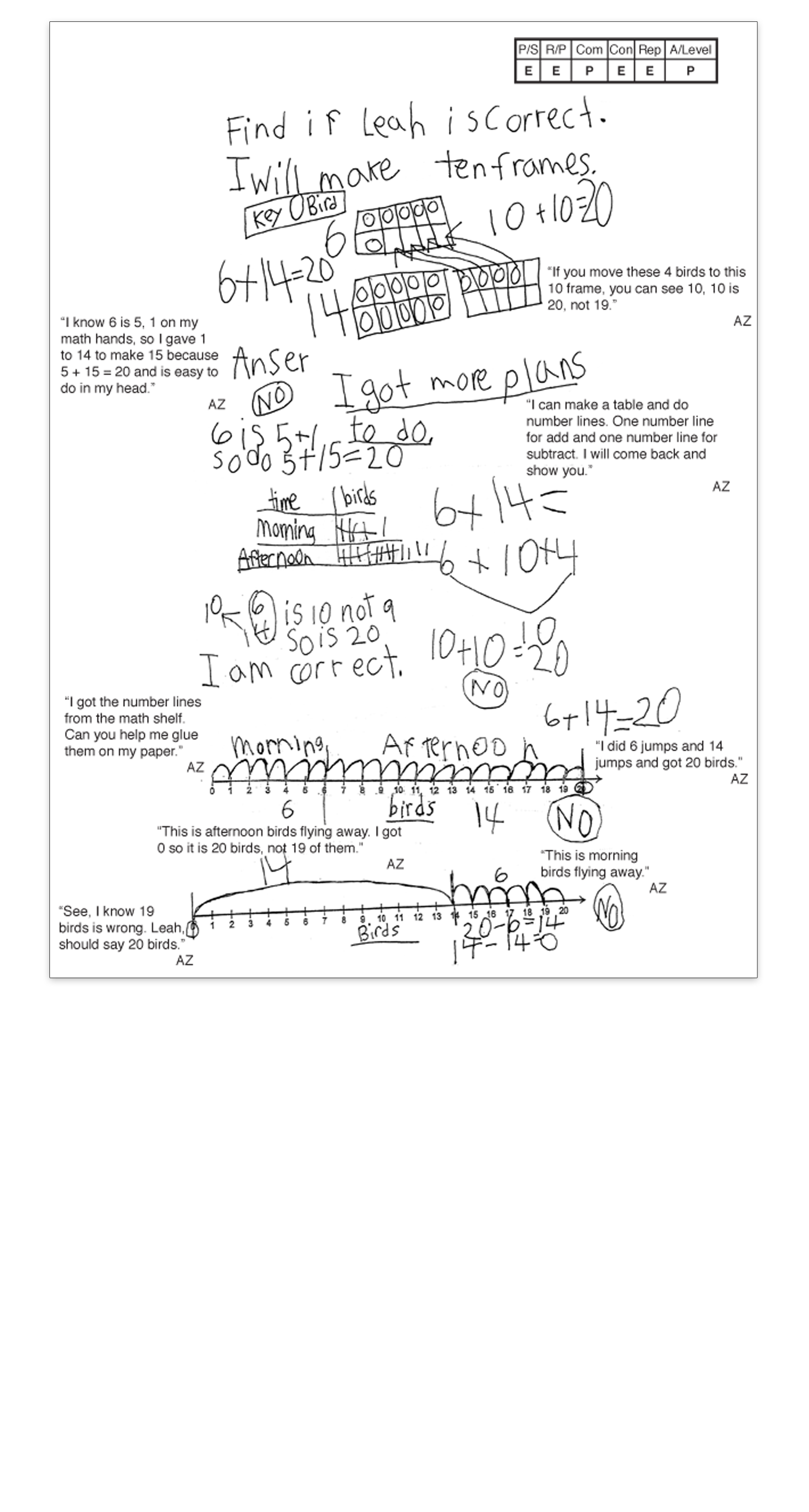2 / 6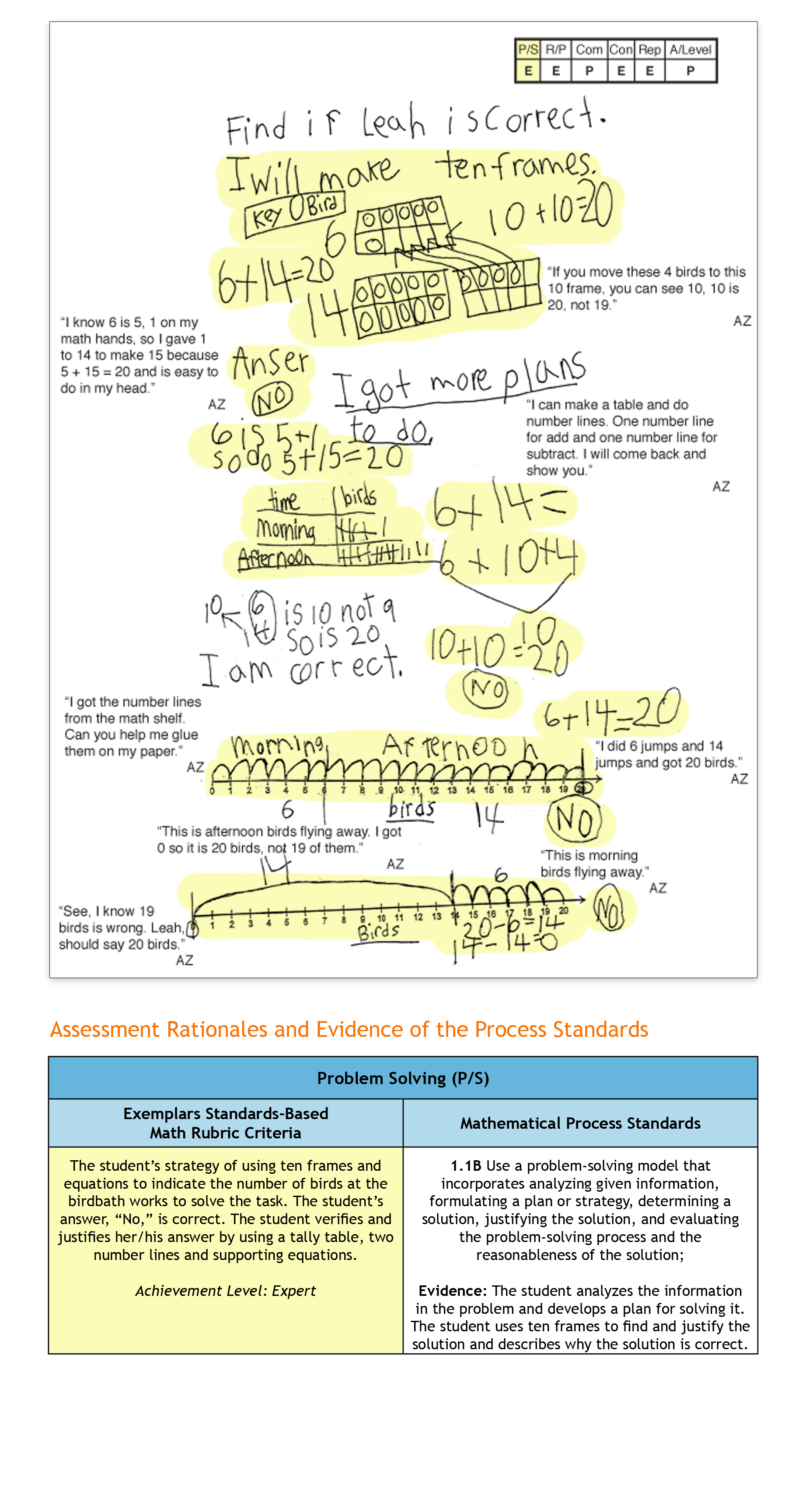3 / 6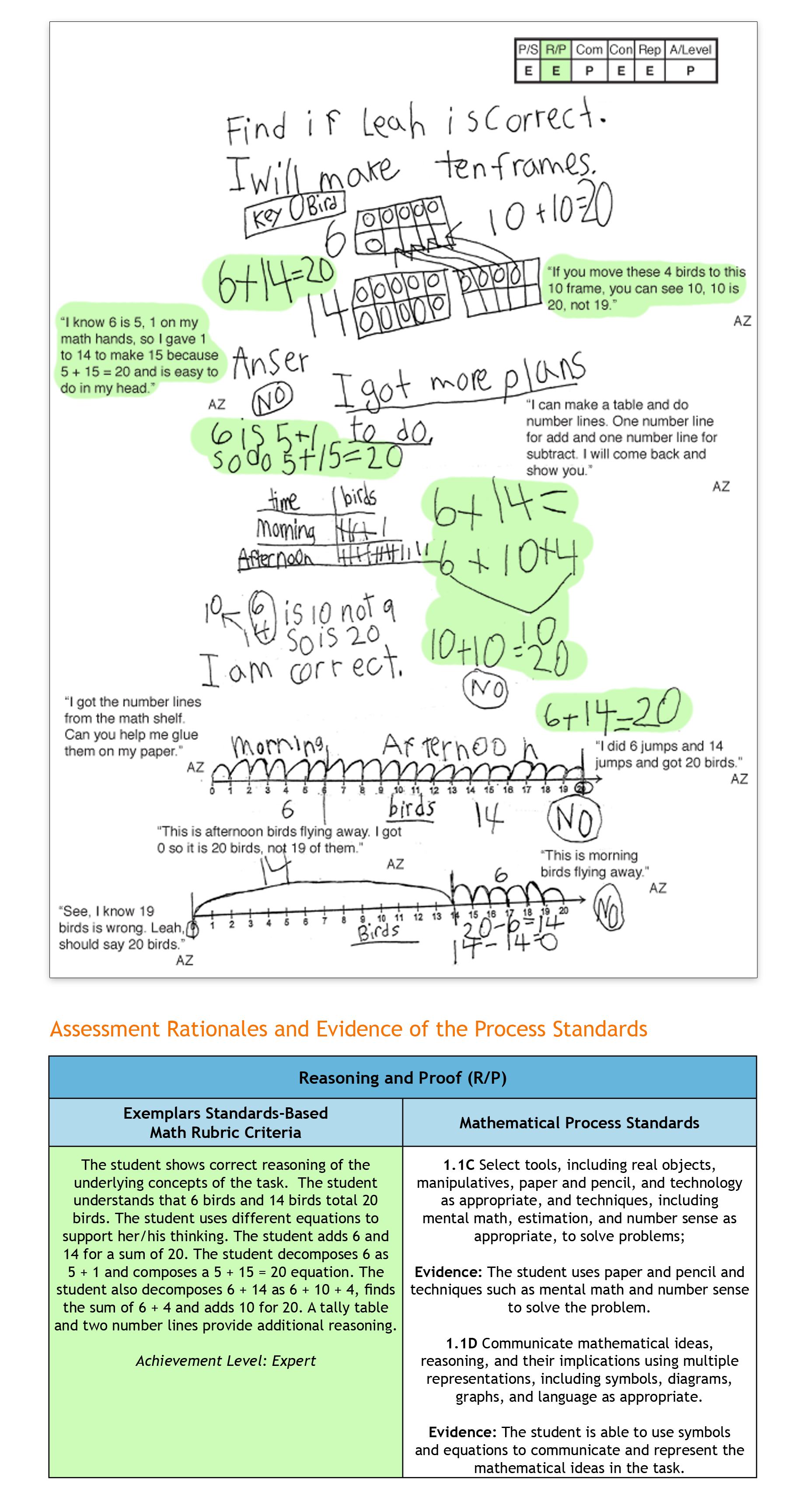4 / 6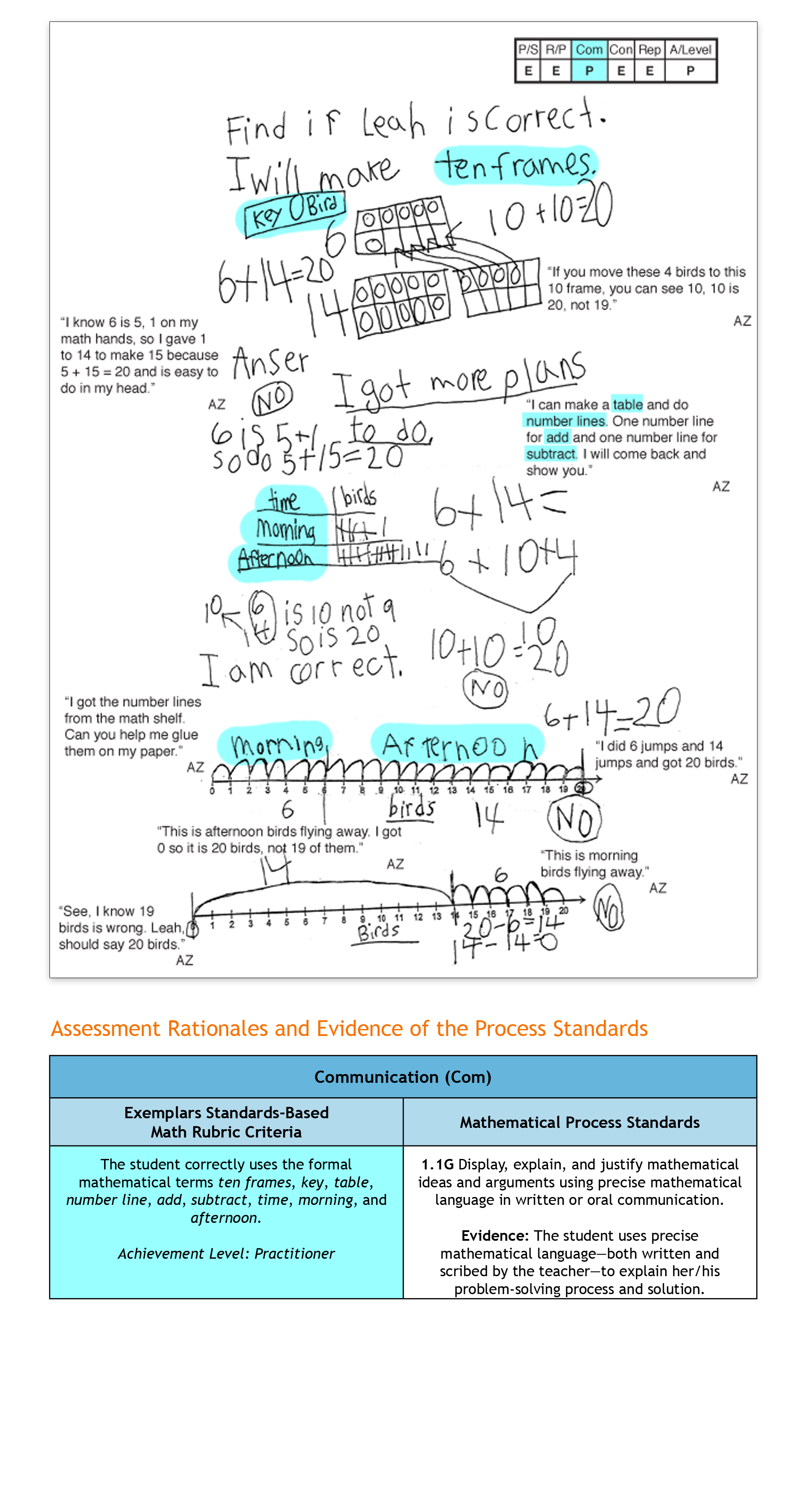5 / 6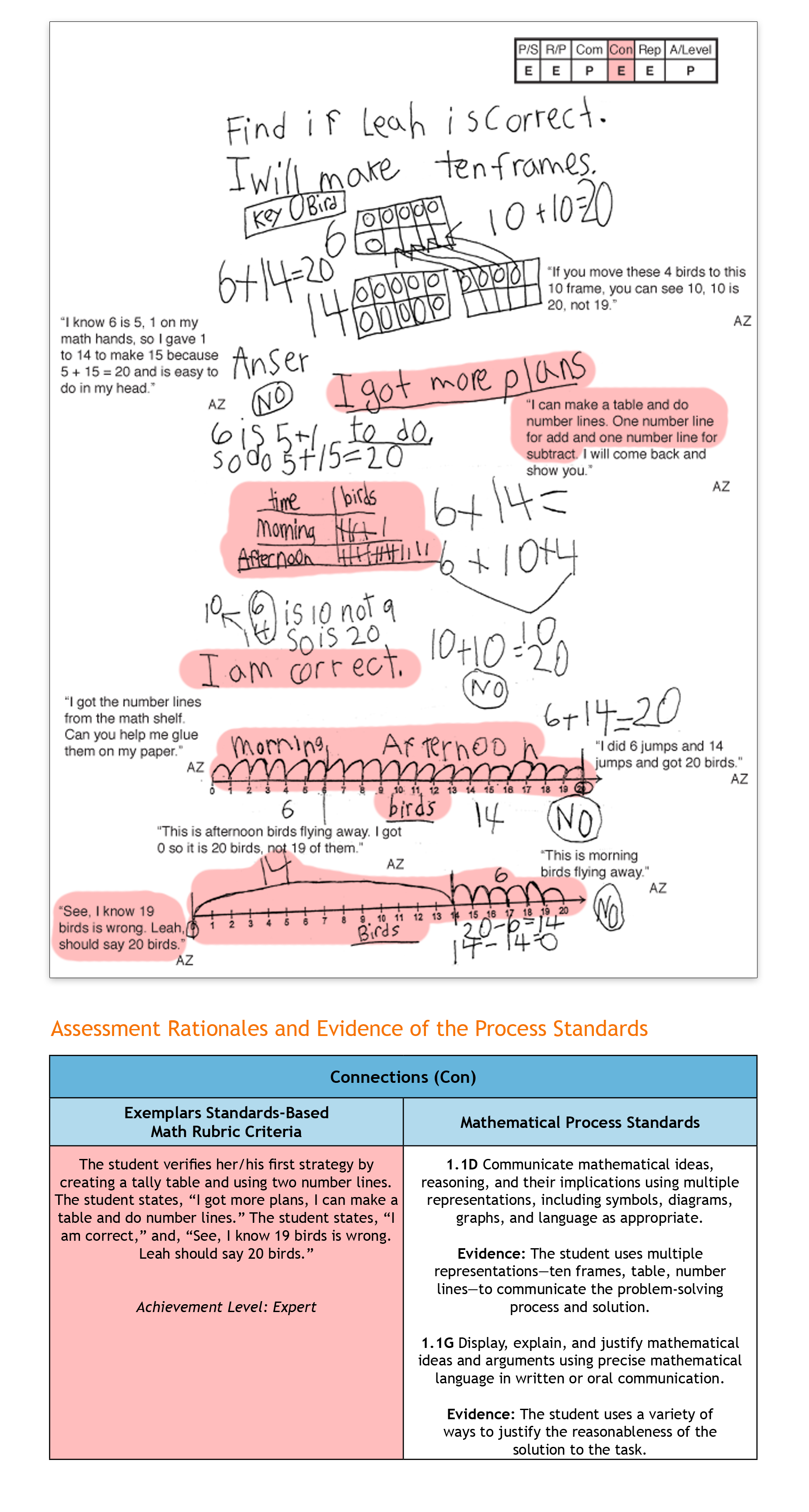6 / 6Topics: